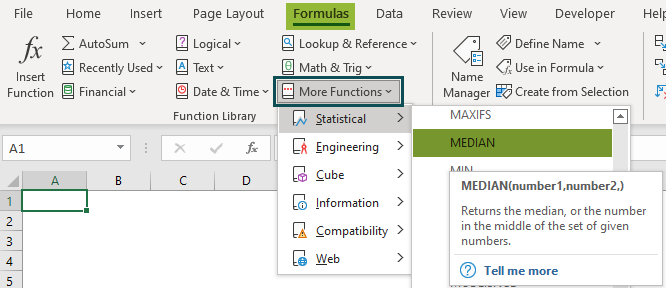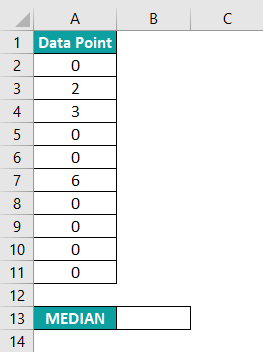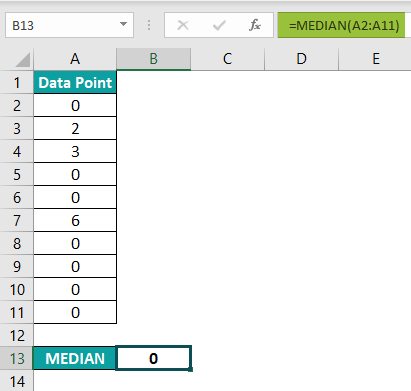# MEDIAN Excel FunctionArticle byExcelMojo Team## What Is MEDIAN Function In Excel?

The MEDIAN function in Excel is an inbuilt Statistical function that returns the median or the value in the middle of the supplied numeric values. Users can use the MEDIAN Excel function to determine median sales or expenditures. And it is more useful when working with skewed data distribution and when the distribution has outliers.

For example, the below table shows different height values in cm.

Suppose we want to determine the median height for the above data set containing height values. Then we can use the MEDIAN Excel function. In the above MEDIAN Excel function example, the data set has nine data points. So the MEDIAN() returns the middle height value, 160 cm, when the data points are in a numeric order, {150, 153, 154, 159, 160, 162, 165, 166, 173}.

Thus, whether the numbers in the given set of values are in the numeric order or random order, the MEDIAN Excel function return value will remain the same.

###### Key Takeaways
• The MEDIAN Excel function determines the median of a given set of numeric values. The function works well for skewed data distribution or when the distribution contains outliers.
• The MEDIAN() takes one mandatory argument, number1, with the subsequent arguments being optional. And the supplied dataset can be in numeric order or random order. In each case, the function considers the dataset in its numeric order to calculate the median value.
• We can use MEDIAN() with other Excel functions, such as IF, involving a single or multiple conditions. But we will have to execute the expression as an array formula using Ctrl + Shift + Enter.

### MEDIAN() Excel Formula

The MEDIAN Excel formula is:

where,

• number1: A set of one or more numeric values for which we need to determine the median.
• number2: The second set of one or more numeric values.

While the first argument is mandatory in the MEDIAN Excel function, the subsequent arguments are optional. And we can enter the arguments as numbers, named ranges, dates, arrays, or references to cells containing numeric values.

Consider the below critical points while using the MEDIAN().

• In Excel 2007 and above, the supplied MEDIAN Excel function arguments can be a maximum of 255.
• Suppose the given dataset has an even number of data points. Then the MEDIAN() output will be the average of the two numeric values at the center of the dataset when the data points are in numeric order.
• Suppose the given dataset has an odd number of data points. Then the MEDIAN() output will be the number in the middle of the dataset when the data points are in numeric order.
• If the supplied dataset contains text, blank cells, or cell references in Excel considers logical values (TRUE and FALSE) entered directly as arguments and cells with zeros while calculating the median value.

### How To Use MEDIAN Excel Function?

The steps to use the MEDIAN Excel Function are:

1. First, ensure the source data set is complete and accurate.
2. Then select the target cell, enter the MEDIAN(), and press Enter.

Let us see a MEDIAN Excel Function example to understand the above steps.

Consider the below table. It shows the number of mobile units sold each weekend in June and July.

As June and July have four and five weekends, their datasets have four and five values, respectively. And here is how we can use the MEDIAN Excel function to determine the median values for the two months.

Step 1: Select the target cell B9, enter the MEDIAN() provided in the Formula Bar in the image below, and press Enter.

Alternatively, we can select cell B9 and click Formulas > More Functions > Statistical > MEDIAN to open the Function Arguments window.

We can enter the required MEDIAN Excel function arguments in the Function Arguments window, which will get updated in the target cell.

And once we click OK in the Function Arguments window, we will see the MEDIAN Excel function return value as the output in the target cell.

Step 2: Select the target cell B10, enter the MEDIAN() provided in the Formula Bar in the image below, and press Enter.

For June, the dataset contained four data points in numeric order. So the MEDIAN() took the two middle values, 1250 and 1365, and returned their average, 1307.5, as the median value for June.

In the case of July, the data set had five data points, but not in numeric order. So, the MEDIAN() listed the numbers in the numeric order {1200, 1320, 1480, 1500, 2000}, and returned the middle value, 1480, as the median for July.

### Examples

Here are a few illustrations to show how the MEDIAN Excel function works.

#### Example 1

Let us see what the Median Excel function returns when a few data points in the dataset are text values.

Consider the below table. It shows the scores of the students who appeared for the mathematics examination. And for those who did not give the test, the score is NA.

Suppose we need to find the median Mathematics score for the given data. Then we can apply the MEDIAN Excel function to get the required data, and the steps are:

Step 1: Select the target cell B14, enter the MEDIAN() provided in the Formula Bar in the image below, and press Enter.

First the MEDIAN() sorts the data points in their numeric order, {70, 80, 86, 89, 95, 98, 100, NA, NA, NA}. As per the definition of the MEDIAN Excel function explained earlier, the function ignores text values. So, in this example, the function does not consider the value NA while calculating the median value. Instead, it takes the seven numbers in column B. And as the number of data points is odd, it returns the middle number as the median value, 89, when the numbers are in their numeric order.

#### Example 2

In this example, we shall see how the MEDIAN() behaves when the data points in the data set are dates.

The below table shows the monthly stock update for an item.

Assume we want to determine the median date and the median stock value for the specific item. We can use the MEDIAN Excel function to get the two values.

Step 1: Select the target cell E1, enter the MEDIAN() provided in the Formula Bar in the image below, and press Enter.

The MEDIAN() considers the dates as numeric values. In the above example, the dataset in column A contains an even number of dates in their numeric order. So the MEDIAN() takes the two middle date values, 30-06-21 and 31-07-21, and returns their average as the median data value, 15-07-21.

Please Note: If the date is in a format such as October 31st, 2021, instead of 31-10-21, the MEDIAN() will ignore it. Thus, the data should be in the proper date format in Excel to ensure the function returns the accurate median value.

Step 2: Select the target cell E2, enter the MEDIAN() provided in the Formula Bar in the image below, and press Enter.

Here again, the number of data points in the dataset in column B is even, 12, with the data points not in their numeric order. So, the MEDIAN() takes the data points in their numeric order, {42000, 49000, 50000, 53000, 53000, 54000, 57000, 59000, 60000, 60000, 61000, 68000}, as the input. It then considers the two middle numbers, 54000 and 57000, and returns their average as the item stock median.

#### Example 3

We can use the MEDIAN Excel function with other Excel functions, such as Excel IF.

The first table in the image below shows the daily wage data of five employees.

And suppose we need to determine their median monthly wages for January and February. Then, we can use the MEDIAN Excel function and IF() to populate the required data in the second table.

Step 1: Select the target cell I2, enter the below formula containing the MEDIAN() and IF(), and press the keys Ctrl + Shift + Enter since it is an array excel formula.

=MEDIAN(IF(\$A\$2:\$A\$21=\$F2,IF(\$C\$2:\$C\$21=\$G2,\$D\$2:\$D\$21)))

Step 2: Drag the fill handle downwards to copy the array formula in cell range H3:H11.

For example, let us see how the formula calculates the February median wage for the employee, Harry Haynes, and displays the output in cell H10.

The multiple IF conditions return the dataset, {20,21}, as Harry Haynes had his duty on two days in February (Refer to rows 18 and 19 in the first table depicted in the below image)

Then the MEDIAN() takes the dataset {20,21} as the input. And as the dataset contains an even number of data points, the function returns and displays the average of 20 and 21 as Harry Haynes’s February median wage, \$20.5, in cell I10.

### Important Things To Note

• The MEDIAN Excel function requires at least one number as input and can take a maximum of 255 arguments.
• We can enter the MEDIAN() arguments as numeric values, named excel ranges, arrays, or references to cells containing numeric values.
• The MEDIAN() returns the average of the two middle numbers of the dataset when the number of data points is even, and the values are in their numeric order. And the function returns the center number of the dataset when the number of data points is odd and the values are in their numeric order.
• The MEDIAN() ignores text values, blank cells, or cell references to logical values. However, the function counts logical values (TRUE and FALSE) provided directly as arguments and cells containing zero values.

Where is MEDIAN function in Excel?

The MEDIAN function in Excel is in the Formulas tab. We should click Formulas > More Functions > Statistical > MEDIAN.Is there a MEDIAN IFS function in Excel?

There is no MEDIAN IFS function in Excel. We will not find an inbuilt function MEDIANIFS() in Excel to calculate the median with multiple conditions.

Instead, we can type a formula containing the MEDIAN() and IF functions with the required multiple conditions. And then, we can execute it as an array formula using Ctrl + Shift + Enter.

Why is MEDIAN not working in Excel?

The MEDIAN is not working in Excel, perhaps due to the following reasons:

1. The data set contains text, blank cells, or the arguments we input are cell references to logical values.
2. We use the MEDIAN() with other functions, such as IF and IFERROR, and do not execute the expression as an array formula using Ctrl + Shift + Enter.

Why is my median 0?

Our median is 0, perhaps because more than half of the data points in the given dataset are zero.

For example, the below table shows 10 data points in a dataset.The median for the above dataset will be 0, as depicted in the image below.The reason is that seven out of the 10 data points are zero. So when the MEDIAN() takes the data points as input, it considers the dataset in the numeric order, {0, 0, 0, 0, 0, 0, 0, 2, 3, 6}.

And since the number of data points is even, the function takes the two center values, the zeros at the 5th and 6th positions, as input. And it returns their average, 0, as the median.